deutsch     english    français     Print

## 10.5 CRYPTOSYSTEMS### INTRODUCTION

The principle of secrecy plays an increasingly important role in our modern world. To protect privacy, but to also maintain confidentiality of important government, industrial, and military information, it is necessary to encrypt data so that, if they fell into the hands of the wrong people, it would be impossible or at least very difficult to find out the original information without the disclosure of the decryption method.
When encoding the original data, they are transformed into encoded data. During decoding the original data are restored. If the original data are written using the letter alphabet, we also speak of plaintext and cryptotext.
The description of the method used for decoding is called key. It can also simply consist of a single number, a number string, or a letter string (a keyword). If the same key is used for encoding and decoding, on also speaks of symmetric-key cryptography, or otherwise of an asymmetric (public-key) cryptographic method.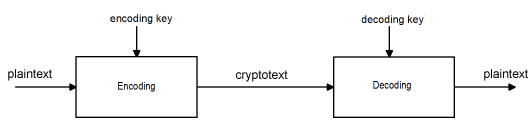PROGRAMMING CONCEPTS: Encoding, decoding, symmetric/asymmetric cryptography, Caesar cipher, Vigenère cipher, RSA encryption, private/public key

### CAESAR CIPHER

According to tradition, Julius Caesar (100 B.C. - 44 B.C.) already applied the following method for his military correspondence: Each plaintext letter is shifted down the alphabet to the right by a certain fixed number of places, continuing on to A again after Z.
 With this method, the alphabet is thus laid out in a ring buffer. With the a shift (key) of 3, the letter A will be encoded in D, B in E, C in F, D in G, etc. You use text files for data in your program so that you can change and share them easily. Write the plaintext using any text editor in the file original.txt that you have to save in the directory where your program is located. Only use capital letters and spaces for the text. You may write multiple lines, so for example: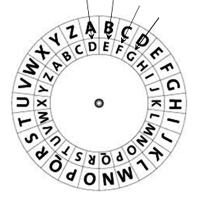TODAY WE MEET AT EIGHT
GREETINGS
TANIA

The encoder encodes the read text string msg from the file using the function encode(msg), where each character is replaced by the corresponding crypto character, except for the line break \n.

```import string
key = 4
alphabet = string.ascii_uppercase + " "

def encode(text):
enc = ""
for ch in text:
if ch != "\n":
i = alphabet.index(ch)
ch = alphabet[(i + key) % 27]
enc += ch
return enc

fInp = open("original.txt")
fInp.close()

print("Original:\n", text)
krypto = encode(text)
print("Krypto:\n", krypto)

fOut = open("secret.txt", "w")
for ch in krypto:
fOut.write(ch)
fOut.close()
```
Highlight program code (Ctrl+C copy, Ctrl+V paste)

XSHEBD IDQIIXDEXDIMKLX
KVIIXMRKW
XERME

The decoder is built analogously, except that the characters in the alphabet are shifted backwards.

```import string
key = 4
alphabet = string.ascii_uppercase + " "

def decode(text):
dec = ""
for ch in text:
if ch != "\n":
i = alphabet.index(ch)
ch = alphabet[(i - key) % 27]
dec += ch
return dec

fInp = open("secret.txt")
fInp.close()

print("Krypto:\n", krypto)
msg = decode(krypto)
print("Message:\n", msg)

fOut = open("message.txt", "w")
for ch in msg:
fOut.write(ch)
fOut.close()
```
Highlight program code (Ctrl+C copy, Ctrl+V paste)

### MEMO

 Keep in mind that you have to retain all empty spaces in the crypto text, even if they are at the beginning or the end of a line. It is clear that the encoding can be cracked easily. A good trick is to try it out for all key numbers 1 to 26.

### ENCODING WITH THE VIGENÈRE METHOD

 You can make Caesar cipher safer by applying a different alphabetic shift on each character of the plaintext. This so-called poly-alphabetic substitution could use any permutation of 27 numbers as a key. There is thus a huge number of possible keys, namely:    27! = 10'888'869'450'418'352'160'768'000'000 ≈ 1027 It is a bit easier to use a keyword that is assigned the list of the corresponding characters in the alphabet, so for example, the key ALICE is assigned to the list [0, 11, 8, 2, 4]. When encoding, the characters of the sequence are then shifted alphabetically to 0, 11, 8, 2, 4 and then repeated at 0, 11,... characters.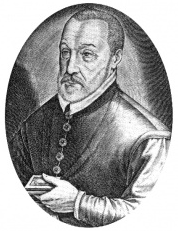Blaise Vigenère (1523-1596)
```import string
key = "ALICE"
alphabet = string.ascii_uppercase + " "

def encode(text):
keyList = []
for ch in key:
i = alphabet.index(ch)
keyList.append(i)
print("keyList:", keyList)
enc = ""
for n in range(len(text)):
ch = text[n]
if ch != "\n":
i = alphabet.index(ch)
k = n % len(key)
ch = alphabet[(i + keyList[k]) % 27]
enc += ch
return enc

fInp = open("original.txt")
fInp.close()

print("Original:\n", text)
krypto = encode(text)
print("Krypto:\n", krypto)
fOut = open("secret.txt", "w")
for ch in krypto:
fOut.write(ch)
fOut.close()
```
Highlight program code (Ctrl+C copy, Ctrl+V paste)

The decoder is again virtually identical.

```import string
key = "ALICE"
alphabet = string.ascii_uppercase + " "

def decode(text):
keyList = []
for ch in key:
i = alphabet.index(ch)
keyList.append(i)
print("keyList:", keyList)
enc = ""
for n in range(len(text)):
ch = text[n]
if ch != "\n":
i = alphabet.index(ch)
k = n % len(key)
ch = alphabet[(i - keyList[k]) % 27]
enc += ch
return enc

fInp = open("secret.txt")
fInp.close()

print("Krypto:\n", krypto)
msg = decode(krypto)
print("Message:\n", msg)
fOut = open("message.txt", "w")
for ch in msg:
fOut.write(ch)
fOut.close()
```
Highlight program code (Ctrl+C copy, Ctrl+V paste)

### MEMO

 The Vigenère encoding method was invented in the 16th century by Blaise de Vigenère and was considered very safe for centuries. If someone knows that the length of the keyword is 5, they must nevertheless try out 265  = 11'881'376 key numbers unless they know something about the word itself, for example that it is the first name of a woman.

### ENCODING WITH THE RSA METHOD

In this method, named after its inventors Rivest, Shamir and Adleman, a key-pair is used, namely a private key and a public key. The original data are encoded with the public key and decoded with the private key. It is an asymmetric cryptographic method.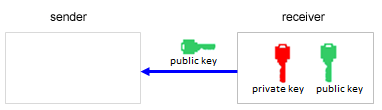Step 1: The receiver generates the private key and the public key and sends the public key to the sender.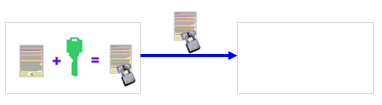Step 2: The sender encodes their message with the public key and sends the encoded text back.Step 3: The receiver decodes the text with the private key.

The keys are generated using the following algorithm based on number theory [more... An elementary proof you can find in the book Barth, Algorithmics for beginners, Springer-Verlag].

First, two prime numbers p and q are chosen, which should have several hundred digits in order for the system to be secure. You multiply these and form m = p*q. From number theory, we know that the Euler function φ(m) = (p-1)*(q-1) is the total of co-prime numbers to m (a, b are co-prime if the largest common factor is ggT(a,b) = 1).

Next, you select a number e that is smaller than φ and co-prime to φ. With this, we already have the public key and it consists of the number pair:

Public key: [m, e]

Below is an example with the small prime numbers p = 73 and q = 151:

m = 73 * 151 = 11023,    φ = 72 * 150 = 10800,    e = 11 (chosen co-prime to φ)

Public key: [m, e] = [11023, 11]

The private key then consists of the number pair:

Private key: [m, d]

where for the number d we must ensure: (d * e) mod φ = 1

(since e and φ are co-prime, Bézout's lemma from number theory says that the equation has to have at least one solution).

You can determine the number d with your values for e and φ using a simple program by trying out 100,000 values for d in a for loop.

```e = 11
phi = 10800

for d in range(100000):
if  (d * e) % phi == 1:
print "d", d
```

You get several solutions (5891, 16691, 27491, 49091, etc.). However, in principle you only need the first solution to determine the private key.

Private key: [m, d] = [11023, 5891]

In this case, calculating the private key is only so easy because you already know the numbers p and q, and hence also the number φ. Without knowing these numbers, the private key can only be calculated with great effort.

The RSA algorithm is used to encode numbers. In order to encode a text, you can use the ASCII code for each character and create the encoded value s with the public key [m, s] for the ciphertext according to the formula:

s = re (modulo m).

Write these encoded numbers line by line in the file secret.txt.

```publicKey = [11023, 11]

def encode(text):
m = publicKey
e = publicKey
enc = ""
for ch in text:
r = ord(ch)
s = int(r**e % m)
enc += str(s) + "\n"
return enc

fInp = open("original.txt")
fInp.close()

print("Original:\n", text)
krypto = encode(text)
print("Krypto:\n", krypto)
fOut = open("secret.txt", "w")
for ch in krypto:
fOut.write(ch)
fOut.close()
```
Highlight program code (Ctrl+C copy, Ctrl+V paste)

In the decoder, you read the numbers from the file secret.txt (first) into a list. In order to decode, you calculate the original number according to the formula below which uses the private key s:

r = sd (modulo m).

This is the ASCII code of the original character.

```privateKey = [11023, 5891]

def decode(li):
m = privateKey
d = privateKey
enc = ""
for c in li:
s = int(c)
r = s**d % m
enc += chr(r)
return enc

fInp = open("secret.txt")
krypto = []
while True:
if line == "":
break
krypto.append(line)
fInp.close()

print("Krypto:\n", krypto)
msg = decode(krypto)
print("Message:\n", msg)
fOut = open("message.txt", "w")
for ch in msg:
fOut.write(ch)
fOut.close()
```
Highlight program code (Ctrl+C copy, Ctrl+V paste)

### MEMO

 The big advantage of the RSA method is that no secret key information has to be exchanged between the sender and the receiver. Rather, the receiver generates both the public and the private key and only communicates the public key to the sender, while keeping the private key secret. The sender can now encode its data, but only the receiver can decode it [more... The transmitter and receiver can be connected also over an insecure channel, such as the Internet. ]. In practice, one could choose very large prime numbers p and q (several hundred digits longs). Generating the public key only requires the product formation m = p * q, which is very simple. If a hacker wants to find out the private key from the public key, they have to inversely determine both secret prime factors of m. Until now, factorizing a long number is only possible with an enormous amount of computational effort. That is how cryptosystems utilize the limits of calculability. In principle, however, there are no absolutely secure encoding methods. Encoding is already considered to be safe once the time it takes to decode is considerably longer than the time during which the information is of importance.

### EXERCISES

 1 Try to decode the ciphertext OAL SHDXTKMJXSASTVVXHLSL XSAFNALTLAGF UMLSASVTFSGFDQSUXSL XJXSTLSXAZ L ZJXXLAFZK XNXDAFX It is a Caesar cipher. Note: One possible solution is to assume that the letter E occurs the most often in English texts. However, you can also try out all shifting possibilities. 2 Educate yourself on the Scytale encoding method from the Internet and implement an encoder/decoder based on its principle. 3 Explain why the Caesar cipher is a special case of the Vigenère method. 4 Generate a public and a private key using two prime numbers p and q (both smaller than 100) according to the RSA method and encode/decode a text.